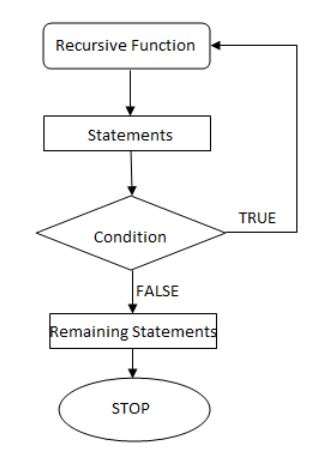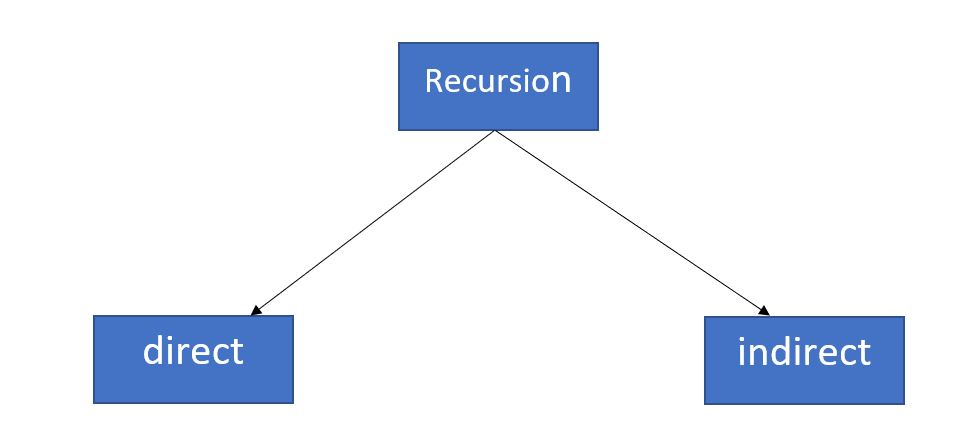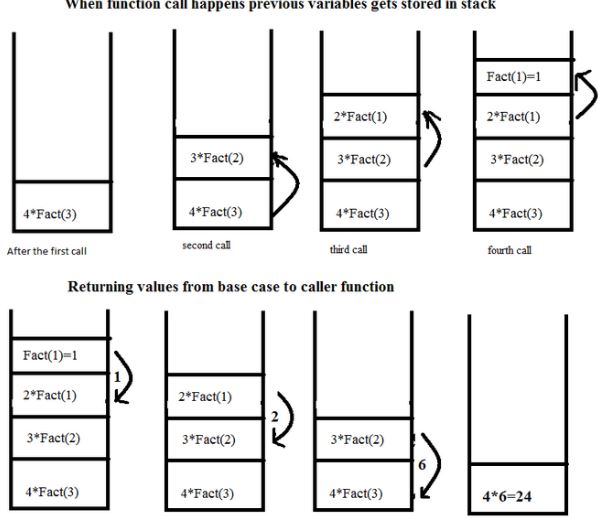×

Search anything:

# An in-depth look into Recursion in C

#### Software Engineering recursion loop C Programming C++Get this book -> Problems on Array: For Interviews and Competitive Programming

Recursion is a coding technique/ design in which a function calls itself directly or indirectly and the corresponding function is called as recursive function. Using this many problems can be solved easily with less time.

Note that to use recursion, the programming language and platform should support this. All major languages including C, C++, Java, Python, Go and others support Recursion. Fortan 77, an early programming language does not support recursion.

There are two major parts in recursion:

• base condition: the condition in which a value is returned and no futher functions are called.
• recursion condition: the way return value of another function call is used to generate the return value of the parent function call

Note that if there is no base condition, then it results in an infinite loop of function calls and the system will halt.

### Flow chart of recursion### What is base condition in recursion?

In recursive program we have 2 conditions to be followed :

1. The solution of bigger problem are reduced into smaller problems or subproblems.
2. The solution is already available.
``````int fact(int n)
{
if (n < = 1) // base case
return 1;
else
return n*fact(n-1);
}
``````

In the above example, base case for n < = 1 ( as the value for n < = 1 is always 1 )is defined and larger value of number can be solved by converting to smaller one till base case is reached.

### Types of recursion

There are two types of recursion:

• Direct recursion
• Indirect recursionDirect Recursion :

A function fun is called direct recursive if it calls the same function fun.

``````void directFun()
{
// Some code....

directFun();

// Some code...
}
``````

Indirect Recursion

A function fun is called indirect recursive if it calls another function say fun_new and fun_new calls fun directly or indirectly.

``````void indirectFun1()
{
// Some code...

indirectFun2();

// Some code...
}
void indirectFun2()
{
// Some code...

indirectFun1();

// Some code...
}
``````

### How recursion works?

Recursive functions use something called “the call stack.” When a program calls a function, that function goes on top of the call stack. This similar to a stack of books. You add things one at a time. Then, when you are ready to take something off, you always take off the top item.

I will show you the call stack in action with the factorial function. factorial(4) is written as 4! and it is defined like this: 4! = 4 * 3 * 2 * 1. Here is a recursive function to calculate the factorial of a number:

``````function fact(x) {
if (x == 1) {
return 1;
} else {
return x * fact(x-1);
}
}
``````

Now let’s see what happens if you call fact(4) The illustration bellow shows how the stack changes, line by line. The topmost box in the stack tells you what call to fact you’re currently on.### Conversion of a loop into recursion

Key points:

• Any loop can be converted into recursion
• Any recursion can be converted into loops like for loop

The following code uses the while loop to compute the Greatest Common Divisor (GCD) of two numbers:

``````unsigned greatest_common_divisor (unsigned a, unsigned b)
{
while (a != b)
{
if (a > b)
{
a -= b;
}
else if (b > a)
{
b -= a;
}
}
}
``````

The above while loop is converted to a recursion in the following code:

``````unsigned greatest_common_divisor (unsigned a, unsigned b)
{
if (a > b)
{
return greatest_common_divisor ( a-b, b );
}
else if (b > a)
{
return greatest_common_divisor ( a, b-a );
}
else // (a == b)
{
return a;
}
}
``````

1. Requires more space as all functions remains in STACK till reaches the base class.
2. It also has greater time requirements because of function calls and return overhead.

### Difference between recursion and iterations

• A conditional statement decides the termination of recursion while a control variable’s value decide the termination of the iteration statement (except in the case of a while loop).

• Infinite recursion can lead to system crash whereas, infinite iteration consumes CPU cycles.

• Recursion repeatedly invokes the mechanism, and consequently the overhead, of method calls. This can be expensive in both processor time and memory space while iteration doesn’t.

• Recursion makes code smaller while iteration makes it longer.

### Question

#### Recursion is similar to which of the following?

loop
switch case
if else
none
Recursion is similar to a loop.#### Harshita Sahai

Maintainer at OpenGenus | Previously Software Developer, Intern at OpenGenus (June to August 2019) | B.Tech in Information Technology from Guru Gobind Singh Indraprastha University (2017 to 2021)

An in-depth look into Recursion in C